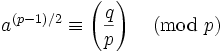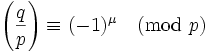## Saturday, January 28, 2006

In today's blog I continue the proof for the Principle of Quadratic Reciprocity. If you are not familiar with the concepts of quadratic reciprocity, quadratic residues, or the Legendre Symbol start here.

If you would like to start this proof at the beginning, start here.

Today's proof is taken from Gareth A. Jones and J. Mary Jones' Elementary Number Theory.

Definition 1: Congruence Class (mod n)

The set of all numbers that are congruent to each other modulo a value n. This congruence class is represented in brackets such as .

NOTE: If you are not familiar with the concept of congruence or modulo, you can review modular arithmetic here.

If we consider n = 3, then we see that:
 = { ... ,-2, 1, 4, 7, ... }

since:

-2 ≡ 4 ≡ 7 ≡ 1 (mod 3).

Definition 2: Unit (mod p)

A congruence class [a] is a unit mod p if there exists another congruence class [b] such that ab ≡1 (mod p).

Here are some examples:

1 is a unit mod 8
3 is a unit mod 8 since (3)(3) ≡ 1 (mod 8)
5 is a unit mod 8 since (5)(5) ≡ 1 (mod 8)
7 is a unit mod 8 since (7)(7) ≡ 1 (mod 8)

Lemma 1: Let p be an odd prime. Let P be the set { 1, 2, ... (p-1)/2 }. Let x,y be elements of P.
Then: x ≠ y → x ± y is not congruent to 0.

(1) Assume that x is less than y (otherwise, we can use the same arguments on y)

(2) Case I: x + y

3 ≤ x + y ≤ (p-1)/2 + (p-1)/2 -1 = (p-1)/2 + (p-1-2)/2 = (2p -4)/2 = p-2.

(3) Case II: x-y

-1 ≥ x-y ≥ 1 - (p-1)/2 = (2 - p + 1)/2 = (-p + 3)/2 = -(p-3)/2

(4) Case III: y-x

1 ≤ y - x ≤ (p-1)/2 - 1 = (p-1-2)/2 = (p-3)/2

QED

Lemma 2: Let p be a prime, let a be an integer such that gcd(a,p)=1. Let P be the set { 1, 2, ... (p-1)/2 }. Then:
x ∈ P, y ∈ P, ax ≡ ± ay → x=y

(1) Assume that ax ≡ ± ay (mod p) and x ≠ y.

(2) ax ± ay ≡ 0 (mod p) [See here for a review of modular arithmetic if needed]

(3) So, p divides a(x ± y)

(4) But p doesn't divide a since gcd(a,p) = 1

(5) So, p divides x ± y [By Euclid's Lemma]

(6) So, x ± y ≡ 0 (mod p)

(7) But x ± y is not congruent to 0 (mod p) from Lemma 1 above.

(8) So we have a contradiction and we reject (#1)

QED

Corollary 2.1: If p is an odd prime and P = { 1, 2, ... (p-1)/2 } and gcd(a,p)=1, then there exists εi where aP = { (ε1), (ε2)(2), ... (ε(p-1)/2)[(p-1)/2] } and each εi = +1 or -1.

(1) We know that each element of aP must be in { ±1, ±2, ... ±(p-1)/2 } since:

(a) Let b be any element of P.

(b) We know that ab must fit into one of the following congruence classes [± 1], [± 2], ... [ ±(p-1)/2 ] since ab (mod p) must be between 0 and p-1.

(c) But this means that either it is between 0 and (p-1)/2 or it is between (p-1)/2 + 1 and p - 1.

(d) If it is between 0 and (p-1)/2 then we are done.

(e) If it is between (p-1)/2 + 1 and p-1, then ab (mod p) is between -(p-1)/2 and -1 since p-1 ≡ -1 (mod p) and since [(p-1)/2 + 1] ≡ -(p-1)/2 (Consider that (p-1)/2 + 1 + (p-1)/2 = (p-1+2 +p - 1)/2 = 2p/2 = p.)

(f) But then either way ab is in { ± 1, ± 2, ... ± (p-1)/2 }

(2) We know that aP = { ±1, ±2, ... ±(p-1)/2 } since:

(a) Let's suppose we have b,c such that b,c ∈ P and b ≠ c.

(b) Then, we know that that ab is not congruent to ac from Lemma 2 since it if they were congruent then they must be the same which contradicts our assumption.

QED

Lemma 3: if p is an odd prime and a is a unit mod p, then a(p-1)/2 ≡ (∏εi) (mod p) where εi is derived from aP as in Corollary 2.1 above.

(1) We know that aP = { ± 1, ± 2, ... ±(p-1)/2 } where at each point εi = 1 or -1 [See corollary 2.1]

(2) (∏εi) ≡ [(a*1)(a*2)...(a*(p-1)/2)]/[(1)(2)...(p-1)/2] (mod p)

NOTE: We can make this assumption since multiplication in modular arithmetic is an abelian group. More details here.

(3) Now, be definition of factorial [(p-1)/2]! ≡ (1)(2)...(p-1)/2 [See here for review of factorials]

(4) Likewise (a*1)(a*2)*...*(a*[p-1]/2) ≡ a(p-1)/2[(p-1)/2]! (mod p)

(5) Combining #2 with #3 and #4 gives us:
(∏εi) ≡ a(p-1)/2[(p-1)/2]!/[(p-1)/2]! = a(p-1)/2 (mod p)

QED

Lemma 4: Gauss's Lemma for Quadratic Residues

If p is an odd prime and gcd(q,p)=1, then:where:
μ = the number of elements in qP ∩ N
P = { 1, 2, ... (p-1)/2 }
N = { -1, -2, ... -(p-1)/2 }

(1) Let x,y be elements of P such that x ≠ y.

(2) qx is not congruent to ± qy (mod p) (from Lemma 2 above)

(3) So, we see from (2) that there are (p-1)/2 distinct congruence classes that are created from qP since for each combination, we know from (2), that there is no overlap.

(4) q(p-1)/2 ≡ (∏εi) (mod p) where εi = 1 if (i) ∈ P and εi = -1 if (i) ∈ N [From Lemma 3 above]

(5) So, q(p-1)/2 ≡ (-1)μ (mod p) where μ = the number of elements in qP ∩ N since:

(a) (∏εi) = (-1)μ where μ is the number of times εi = -1. [Since we can ignore all the times εi = 1]

(b) But this means we need to figure out the count of all elements b ∈ qP that are also in N.

(c) And this means that we are looking for the number of elements in qP ∩ N.

(6) By Euler's Criterion (see here), we know that:(7) Combining (5) and (6) gives us our conclusion since the Legendre symbol is only = to 1 or -1.QED

#### 1 comment:Anonymous said...

x +/- y = 0 (mod p) is not the same as saying x = y (mod p) in Lemma 2

but Z= {1,...,n-1/2} and x and y belong to Z. x + y = v (mod p)

v will never equal 0.